# Current Electricity

## Exercise 9(A)

#### Question 1

Name one d.c. source and one a.c. source.

The cell or a battery is a source of direct current (d.c.).

The mains in our house or an a.c. generator are the source of alternating current (a.c.).

#### Question 2

Distinguish between d.c. and a.c.

The differences between d.c. and a.c are as follows —

Direct current (d.c.)Alternating current (a.c.)
Direct current (d.c) is a current of constant magnitude flowing in one directionAlternating current (a.c.) is the current for which both the magnitude and direction change with time.
The cell or a battery is a source of d.c. current.The mains in our house or an a.c. generator are the source of alternating current (a.c.).

#### Question 3

What is an electric cell?

A cell basically consists of a vessel containing two conducting rods, called the electrodes, at some separation, placed or immersed in a solution (or jelly), called the electrolyte.

In a cell, chemical energy changes into electrical energy when it sends current in a circuit. The below figure shows a light bulb connected to an electric cell: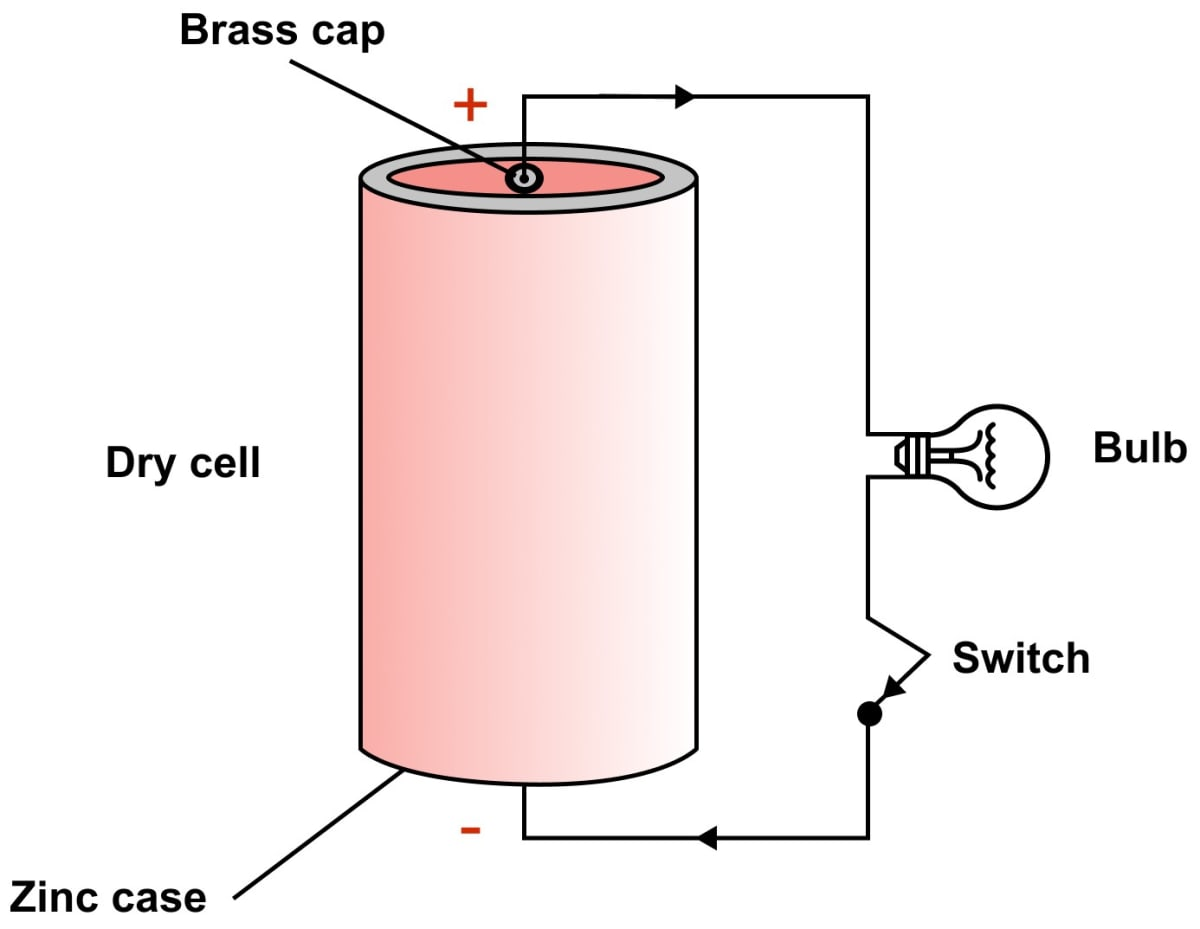#### Question 4

What transformation of energy takes place when current is drawn from a cell?

When current is drawn from a cell, chemical energy changes to electrical energy.

#### Question 5

Name the constituents of a cell.

A cell basically consists of a vessel containing two conducting rods, called the electrodes, at some separation, placed or immersed in a solution (or jelly), called the electrolyte.

#### Question 6

State the two kinds of cell. Give one example of each.

The two kinds of cells are as —

1. Primary cell — These cells provide current as a result of irreversible chemical reaction.
Example: Daniel cell is an example of a primary cell.
2. Secondary cells or accumulators — In these cells chemical reaction is reversible and so they can be recharged after use.
Example: Ni-Fe (or alkali) accumulator is an example of a secondary cell.

#### Question 7

What is a primary cell? Name two such cells.

Primary cell — These cells provide current as a result of irreversible chemical reaction. The cells are discarded after use when the entire chemical energy in them has been converted into electrical energy. Thus, these are 'use and throw' types of cells and cannot be recharged.

Depending upon the material of electrodes and electrolyte, we have different types of primary cells e.g., simple voltaic cell, dry cell.

#### Question 8

What is a secondary cell? Name one such cell.

Secondary cells or accumulators also provide current as a result of a chemical reaction. In these cells, the chemical reaction is reversible and so they can be recharged after use. e.g., Lead accumulator.

#### Question 9

State three differences between primary and secondary cells.

The differences between a primary and a secondary cell are —

Primary cellSecondary cell
Chemical reactions are irreversible.Chemical reactions are reversible.
Chemical energy is converted into electrical energy when current is drawn from it.Electrical energy converts into chemical energy when current is passed in it (i.e., during charging), while chemical energy converts into electrical energy when current is drawn from it (i.e., during discharging).
It can not be recharged.It can be recharged.

#### Question 10

What do you understand by the term current? State and define it's S.I. unit.

Current is the rate of flow of charge across a cross-section normal to the direction of flow of current.

The S.I. unit of current is coulomb per second which is called ampere. It is denoted by the symbol A.

#### Question 11

How much is the charge of an electron?

The charge on an electron is -1.6 x 10-19 coulomb.

#### Question 12

n electrons flow through a cross section of a conductor in time t. If charge on an electron is e, write an expression for the current in the conductor.

If n electron pass through the cross section of a conductor in time t and charge on an electron is e, then

Total charge passed Q = n x e

and current in conductor I = $\dfrac{Q}{t}$ = $\dfrac{ne}{t}$

#### Question 13

Name the instrument used to control current in an electric circuit.

The instrument used to control current in an electric circuit is a Rheostat. It can adjust the magnitude of current in a circuit by changing the length of the resistance wire in the circuit.

#### Question 14

In the electric circuit shown in the figure below, label the parts A, B, C, D, E, and F. State the function of each part. Show in the diagram the direction of flow of current.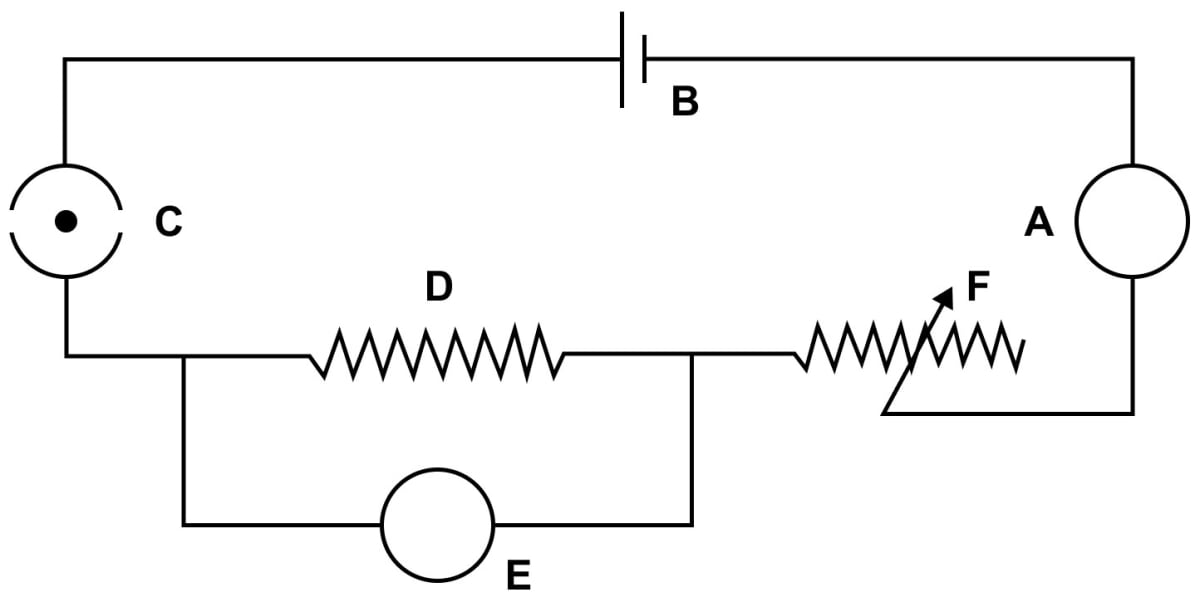The diagram below shows the direction of flow of current marked by red arrows and the labelled parts — A, B, C, D, E, and F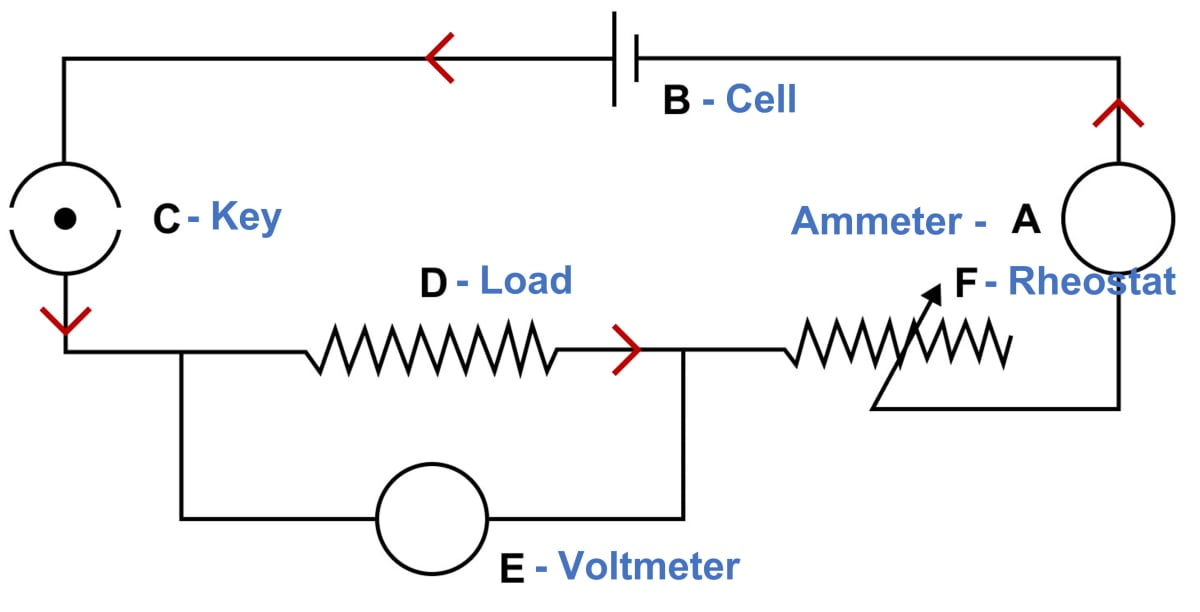The name and function of each part is as follows —

A → Ammeter.

An ammeter is an instrument used to measure the magnitude of current flowing in a circuit.

B → Cell.

It acts as a source of direct current for the circuit.

C → key.

It is used to put the current on and off in the circuit.

An appliance which is connected in a circuit. It may be a resistance (e.g., bulb, heater etc.) or a combination of different electrical components.

E → Voltmeter.

A voltmeter is used to measure the potential difference between two points of a circuit.

F → Rheostat.

A rheostat is a device by which resistance in a circuit can be varied continuously.

#### Question 15

What is the function of a key (or switch) in an electric circuit?

A key is used to put the current on and off in a circuit. When key is open (i.e., the plug is taken out), the circuit is incomplete. When key is closed (i.e., the plug is put in) current flows through the circuit and the circuit is said to be closed.

#### Question 16

Write symbols and state functions of each of the following components in an electric circuit —

(i) key

(ii) cell

(iii) rheostat

(iv) ammeter

(v) voltmeter

The symbol and function of the components are as follows —

(i) Key

Symbol: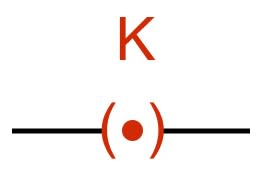Function:
A key is used to put the current on and off in a circuit.

(ii) Cell

Symbol: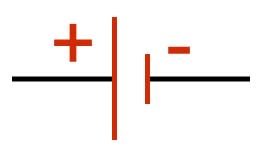Function:
A cell acts as a source of direct current for the circuit.

(iii) Rheostat

Symbol: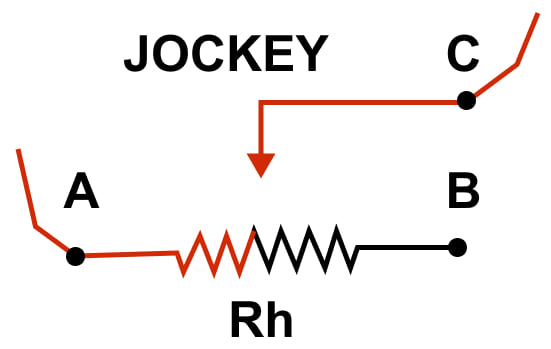Function:
It controls the flow of current in a circuit.

(iv) Ammeter

Symbol: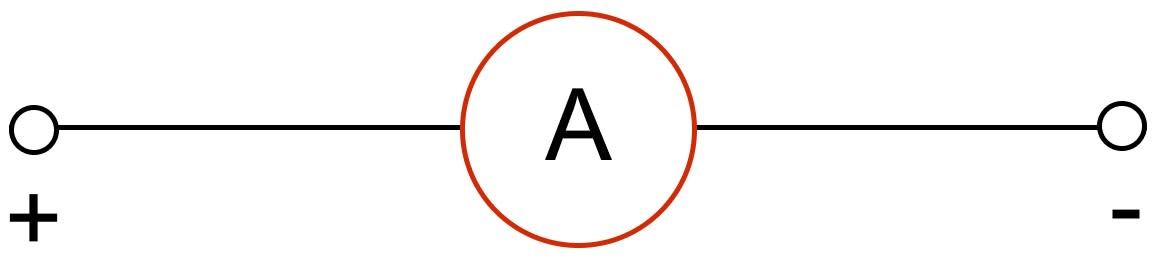Function:
An ammeter is an instrument used to measures the magnitude of current flowing in a circuit.

(v) Voltmeter

Symbol: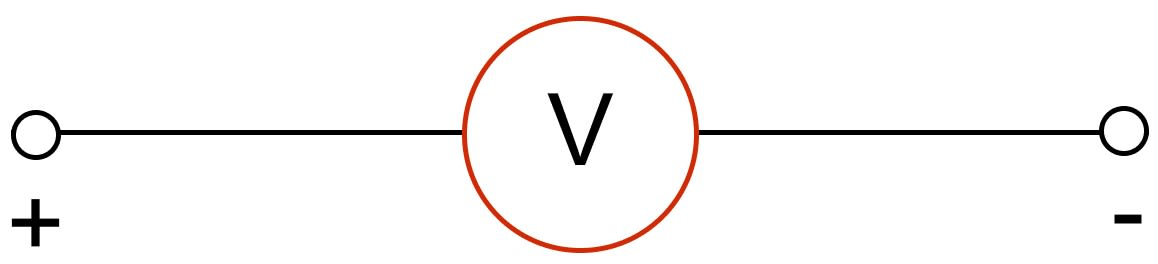Function:
A voltmeter is used to measure the potential difference between two points of a circuit.

#### Question 17

(a) Complete the circuit given in figure by inserting between the terminals A and C, an ammeter. (b) In the diagram mark the polarity at the terminals of ammeter and indicate clearly the direction of flow of current in the circuit, when the circuit is complete. (c) Name and state the purpose of Rh in the circuit.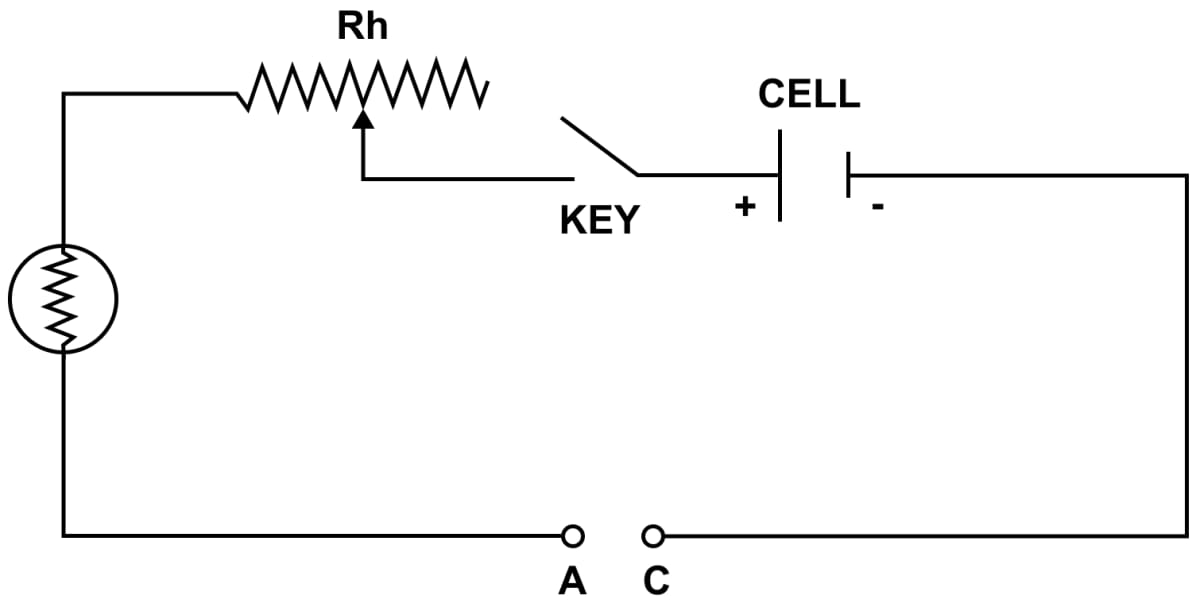(a) The circuit with ammeter between the terminals A and C with polarity marked is shown below: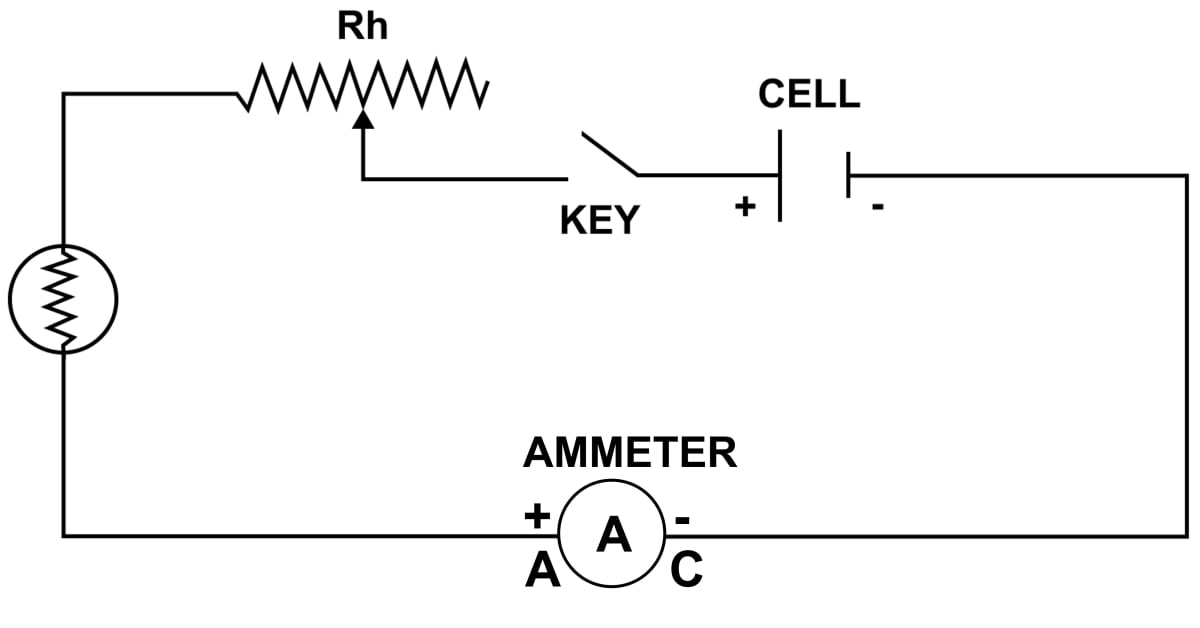(b) The direction of flow of current in the completed circuit with ammeter (polarity marked) is shown below: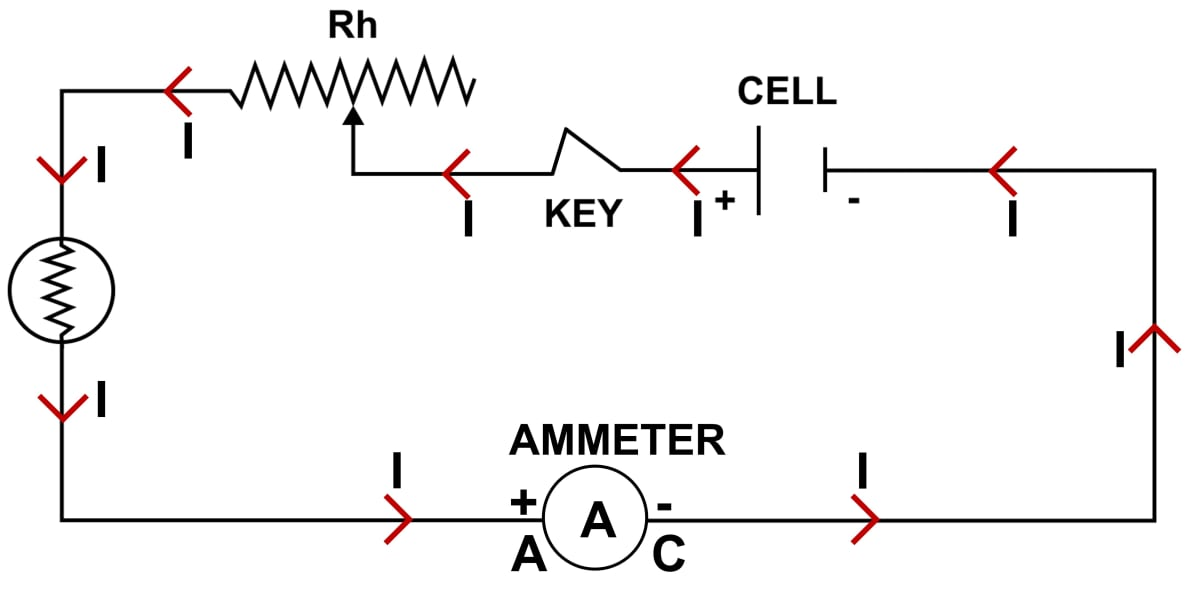(c) Rh is the rheostat. It controls the flow of current in a circuit.

#### Question 18

What are conductors and insulators of electricity? Give two examples of each.

Conductors — The substances which allow the current to flow through them easily are called conductors. They have large number of free electrons and they offer a very small resistance to the flow of current.

Examples — impure water (or acidulated water) and mercury are conductors of electricity.

Insulators — The substances which do not allow current to flow through them are called insulators. They have almost no electrons and offer a very high resistance to the flow of current.

Example — cotton, rubber.

#### Question 19

Select conductors of electricity from the following — Copper wire, silk thread, pure water, acidulated water, human body, glass, mercury.

The conductors of electricity are — copper wire, acidulated water, human body and mercury.

#### Question 20

State two differences between a conductor and an insulator of electricity.

The differences between a conductor and an insulator of electricity are as follows —

ConductorInsulator
Allow the current to flow through them easilyDo not allow current to flow through them.
They have large number of free electrons and they offer a very small resistance to the flow of current.They have almost no electrons and offer a very high resistance to the flow of current.

#### Question 21

Distinguish between a closed circuit and an open circuit, with the use of suitable labelled diagram.

The differences are —

Closed circuitOpen circuit
Current flows only in a closed circuitCurrent does not flow in an open circuit.
For an electric circuit to be closed, each component of it must pass current through it, i.e., it should be conductingIf there is an insulator in the path (or the circuit is broken), the circuit is incomplete (or open) and the current will not flow through it.

The diagram below shows closed and open circuits: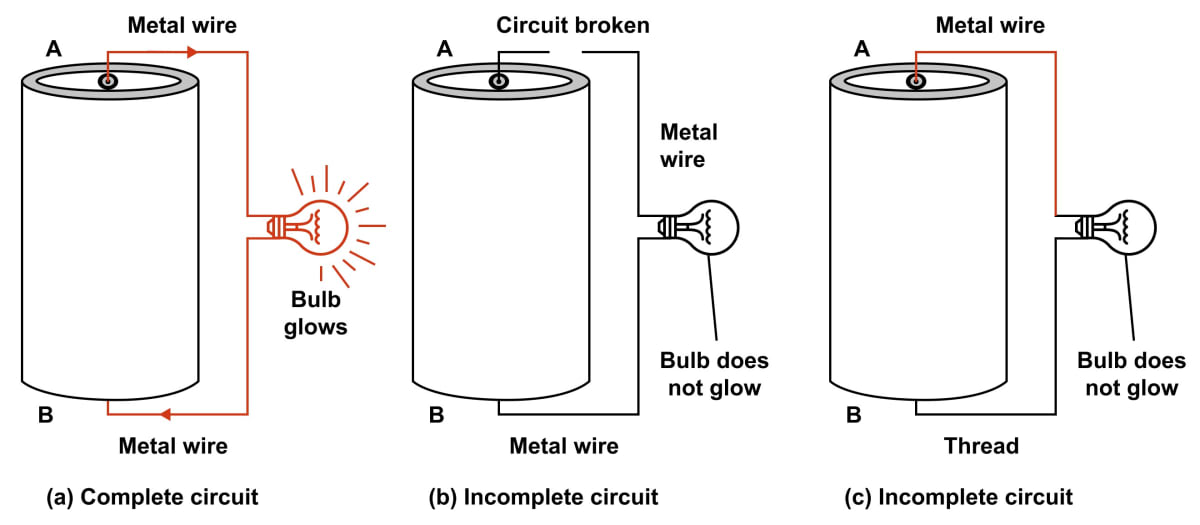#### Question 22

Write the condition required for a circuit to be a closed circuit.

For an electric circuit to be closed, each component of it must pass current through it, i.e., it should be conducting

## Multiple Choice Type

#### Question 1

A cell is used to:

1. measure current in a circuit
2. provide current in a circuit ✓
3. limit current in a circuit
4. prevent current in a circuit

The cell or a battery is a source of direct current (d.c.). It provides current as a result of irreversible reaction.

#### Question 2

The unit of current is:

1. ampere ✓
2. volt
3. ohm
4. coulomb

The S.I. unit of current is coulomb per second which is called ampere. It is denoted by the symbol A.

#### Question 3

The insulator of electricity is:

1. copper
2. acidulated water
3. human body
4. silk ✓

Silk does not conduct electricity, hence is an insulator.

## Numericals

#### Question 1

A charge 0.5 C passes through a cross section of a conductor in 5 s. Find the current.

Given,

charge (Q) = 0.5 C,

time (t) = 5 s

current (I) = ?

$I = \dfrac{Q}{t}$

Substituting the values we get,

$I = \dfrac{0.5}{5} \\[0.5em] \Rightarrow I = 0.1 \text { A}$

Hence, current = 0.1 A

#### Question 2

A current of 1.5 A flows through a conductor for 2.0 s. What amount of charge passes through the conductor?

Given,

current (I) = 1.5 A

time (t) = 2 s

charge (Q) = ?

$I = \dfrac{Q}{t}$

Substituting the values we get,

$1.5 = \dfrac{Q}{2} \\[0.5em] \Rightarrow Q = 1.5 \times 2 \\[0.5em] \Rightarrow Q = 3 \text { C}$

Hence, amount of charge that passes through the conductor = 3 C

#### Question 3

When starter motor of a car is switched on for 0.8 s, a charge 24 C passes through the coil of the motor. Calculate the current in the coil.

Given,

Time = 0.8 s

charge = 24 C

current = ?

$I = \dfrac{Q}{t}$

Substituting the values we get,

$I = \dfrac{24}{0.8} \\[0.5em] \Rightarrow I = 30 \text { A}$

Hence, the current in the coil = 30 A

## Exercise 9(B)

#### Question 1

Figure below shows two conductors A and B. Their charges and potentials are given in the diagram. State the direction of (i) flow of electrons, and (ii) flow of current, when both the conductors are joined by a metal wire.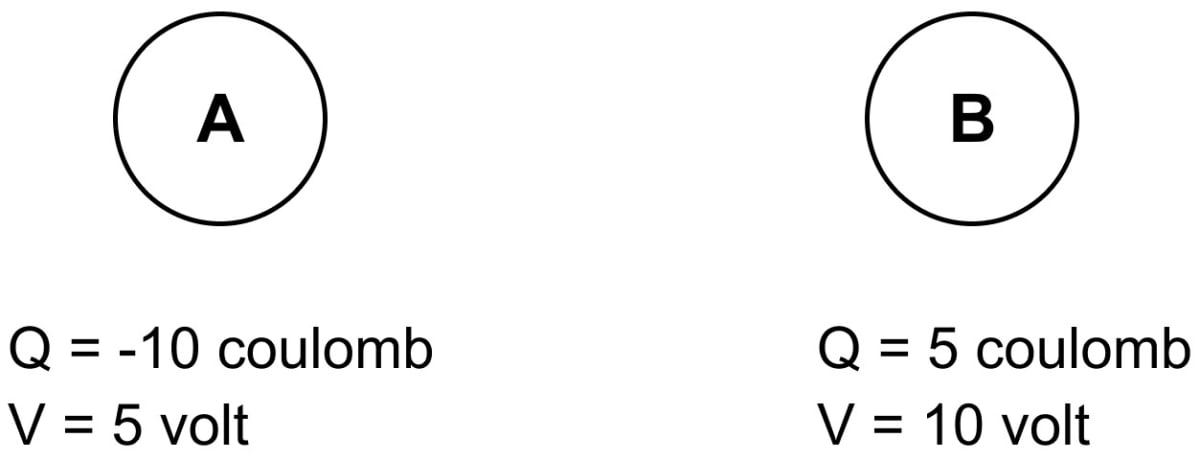(a) The direction of flow of electrons is from A to B (i.e., from a low potential to a high potential)

(b) The direction of flow of current when both the conductors are joined by a metal wire is from B to A. (i.e., from a high potential to a low potential)

#### Question 2

How is the direction of flow of current between two charged conductors determined by their potentials?

The direction of flow of current is from a body at a higher potential to the one at a lower potential i.e., in direction opposite to the direction of flow of electrons.

#### Question 3

Explain the concept of electric potential difference in terms of work done in transferring the charge.

We know that, like charges repel and unlike charges attract, therefore to create an excess or deficit of electrons at a point, some work is to be done in moving the charges (or electrons) against the forces between them. The force between the two charges is zero when they are at infinite separation.

Hence, quantitatively, potential at a point is measured in terms of work done in bringing a charge q from infinity to that point. If work W' is done in bringing a charge q from infinity to a point, then potential at that point is:

$V = \dfrac{W'}{q}$

Hence, the potential difference between two conductors is measured in terms of work done in transferring the charge from one conductor to the other, through a metallic wire.

Potential difference is a scalar quantity.

#### Question 4

Define the term potential difference.

Potential difference between two conductors is equal to the work done in transferring a unit positive charge from one conductor to the other conductor.

If work W is done in transferring a test charge q from one conductor to the other, the potential difference between them is

$V_1 - V_2 = \dfrac{W}{q}$

Potential difference is a scalar quantity.

#### Question 5

State and define the S.I. unit of potential difference.

Potential difference is measured in joule per coulomb which is named as volt (V).

From relation:

$V = \dfrac{W}{q} \\[1em] \text{Unit of potential difference} = \dfrac{\text{unit of work}}{\text{unit of charge}} \\[0.5em] \therefore \text{1 volt} = \dfrac{\text{1 joule}}{\text{1 coulomb}} \\[0.5em] \text{or} \\[0.5em] \text{1 V} = \dfrac{\text{1 J}}{\text{1 C}}$

Hence, the potential difference between two points is said to be 1 volt if work done in transferring 1 coulomb of charge from one point to the other point is 1 joule.

#### Question 6

'The potential difference between two conductors is 1 volt'. Explain the meaning of this statement.

The statement 'potential difference between two conductors is 1 volt' means that 1 joule of work is done in transferring 1 coulomb of charge from one conductor to the other.

#### Question 7

What do you understand by the term resistance?

The obstruction offered to the flow of current by a conductor is called it's electrical resistance.

Resistance is a scalar quantity.

#### Question 8

Explain why does a metal wire when connected to a cell offer resistance to the flow of current.

A metal wire has free electrons which move in a random manner in the absence of any cell connected across it.

When the ends of the wire are connected to a cell, the electrons start moving from the negative terminal of cell to it's positive terminal through the metal wire.

During their movement, they collide with the fixed positive ions and other free electrons of the wire due to which their speed decreases and direction of motion changes.

After each collision, they again accelerate towards the positive terminal and suffer collision with other positive ions and free electrons again. This process continues.

As a result, the electrons do not move in bulk with increasing speeds from one end to the other but they drift towards the positive terminal. This is how a wire offers resistance to the flow of electrons (or current) through it.

#### Question 9

State and define the S.I. unit of resistance.

S.I. unit of resistance is volt per ampere which is named as ohm (Ω).

From relation:

$\text{R} = \dfrac{V}{I} \\[1em] \text{Unit of resistance} = \dfrac{\text{unit of potentail difference}}{\text{unit of current}} \\[0.5em] \therefore \text{1 ohm} = \dfrac{\text{1 volt}}{\text{1 ampere}} \\[0.5em] \text{or} \\[0.5em] \text{1 Ω} = \dfrac{\text{1 V}}{\text{1 A}}$

Hence, the resistance of a conductor is said to be 1 ohm if a current of 1 ampere flows through it when the potential difference across it's ends is 1 volt.

#### Question 10

State Ohm's law.

Ohm's law states that current flowing through a conductor is directly proportional to the potential difference applied across it's ends provided it's temperature is constant.

If a current I flows through a wire when potential difference across the ends of the wire is V, the resistance offered by the wire to the flow of current is the ratio of potential difference across it to the current flowing in it i.e.,

$\text{Resistance of wire (R)} \\[0.3em] = \dfrac{\text{Potentail difference across wire (V)}}{\text{Current flowing in wire (I)}} \\[0.5em] \therefore \text{R} = \dfrac{\text{V}}{\text{I}} \\[0.5em] \text{or} \\[0.5em] \text{V} = \text{I } \text{R}$

#### Question 11

How are the potential difference (V), current (I) and resistance (R) related?

The relation between potential difference (V), current (I) and resistance (R) is —

V = IR

The above relation is also known as Ohm's law, which states that current flowing through a conductor is directly proportional to the potential difference applied across it's ends provided it's temperature is constant.

#### Question 12

'The resistance of wire is 1 ohm'. Explain the meaning of this statement.

'The resistance of wire is 1 ohm' means that current of 1 ampere flows through it when the potential difference across it's ends is 1 volt.

#### Question 13

How is the current flowing in a conductor changed if the resistance of conductor is doubled keeping the potential difference across it the same?

According to Ohm's law,

$\text{V} = \text{I}\text{R} \\[0.5em] \Rightarrow \text{I} = \dfrac{V}{R} \\[1em]$

Let the doubled resistance be 2R. Potential difference remains the same

$\text{I}_{\text{new}} = \dfrac{V}{2R} \\[0.5em] \Rightarrow \text{I}_{\text{new}} = \dfrac{I}{2}$

∴ If the resistance of conductor is doubled keeping the potential difference across it the same then current flowing in a conductor will be halved.

#### Question 14

State three factors on which the resistance of a wire depends. Explain how does the resistance depend on the factors stated by you.

The factors on which the resistance of a wire depend are —

1. The length of the wire — The number of collisions suffered by the moving electrons will be more if they have to travel a longer distance in a wire, therefore a long wire offers more resistance than a short wire (i.e., resistance of a wire is directly proportional to the length of the wire).
2. The area of cross section of wire — In a thick wire, electrons get a larger area of cross section to flow as compared to a thin wire, therefore, a thick wire offers less resistance (i.e., resistance of wire is inversely proportional to the area of cross section of the wire).
3. The temperature of the wire — If the temperature of the wire increases, ions in it vibrate more violently. As a result, the number of collisions increases and hence the resistance of wire increases (i.e., the resistance of the wire increases with the increase in it's temperature.

#### Question 15

How is the resistance of a wire affected if it's (a) length is doubled, (b) radius is doubled?

(a) If the length is doubled, the resistance of the wire doubles because the number of collisions suffered by the moving electrons will be more if they have to travel a longer distance in a wire and that will increase the resistance offered by the wire (i.e., resistance of a wire is directly proportional to the length of the wire).

(b) The resistance of a wire becomes less or one-fourth when the radius of the wire is doubled. As we know, in a thick wire, electrons get a larger area of cross section to flow as compared to a thin wire, therefore, a thick wire offers less resistance (i.e., resistance of wire is inversely proportional to the area of cross section of the wire).

#### Question 16

State whether the resistance of filament of a bulb will decrease, remain unchanged or increase when it glows.

The resistance of the filament increases, when a bulb glows as the temperature of the filament increases.

#### Question 17

Name the physical quantities of which the units are —

(i) volt
(ii) coulomb
(iii) ohm
(iv) ampere

The physical quantities are —

(i) volt — potential difference
(ii) coulomb — charge
(iii) ohm — resistance
(iv) ampere — current

## Multiple Choice Type

#### Question 1

Current in a circuit flows:

1. in direction from high potential to low potential ✓
2. in direction from low potential to high potential
3. in direction of flow of electrons
4. in any direction

Electric current flows from a body at higher potential to a body at lower potential, in a direction opposite to the direction of flow of electrons.

#### Question 2

The unit of potential difference is:

1. ampere
2. volt ✓
3. ohm
4. coulomb

Potential difference is measured in joule per coulomb which is named as volt (V).

From relation V = $\dfrac{W}{q}$

Unit of potential difference = $\dfrac{\text{unit of work}}{\text{unit of charge}}$

The S.I. unit of work is joule and that of charge is coulomb (C), so potential is measured in joule per coulomb which is named as volt.

Hence, the potential difference between two points is said to be 1 volt if work done in transferring 1 coulomb of charge from one point to the other point is 1 joule.

#### Question 3

On increasing the resistance in a circuit, the current in it:

1. decreases ✓
2. increases
3. remains unchanged
4. nothing can be said

Current (I) is inversely proportional to the resistance (R) hence it decreases.

## Numericals

#### Question 1

In transferring 1.5 C charge through a wire, 9 J of work is done. Find the potential difference across the wire.

Given,

charge (q) = 1.5 C

work = 9 J

potential difference across the wire

$V = \dfrac{w}{q}$

Substituting the values in the formula, we get,

$V = \dfrac{9}{1.5} \\[0.5em] \Rightarrow V = 6 V$

Hence, the potential difference across the wire = 6 V.

#### Question 2

A cell of potential difference 12V is connected to a bulb. The resistance of filament of bulb when it glows, is 24Ω. Find the current drawn from the cell.

Given,

V = 12 V

R = 24 ?

I = ?

From Ohm's Law,

V = IR

Substituting the values in the formula, we get,

$12 = I \times 24 \\[0.5em] \Rightarrow I = \dfrac{12}{24} \\[0.5em] \Rightarrow I = 0.5 A$

Hence, the current drawn from the cell = 0.5 A

#### Question 3

A bulb draws current 1.5 A at 6.0 V. Find the resistance of filament of bulb while glowing.

Given,

I = 1.5 A

Potential difference = 6 V

R = ?

From Ohm's Law,

V = IR

Substituting the values in the formula, we get,

$6 = 1.5 \times R \\[0.5em] \Rightarrow R = \dfrac{6}{1.5} \\[0.5em] \Rightarrow R = 4Ω$

Hence, the resistance of filament of bulb while glowing = 4Ω

#### Question 4

A current 0.2 A flows in a wire of resistance 15Ω. Find the potential difference across the ends of the wire.

Given,

I = 0.2 A

R = 15Ω

Potential difference = ?

From Ohm's Law,

V = IR

Substituting the values in the formula, we get,

V = 0.2 x 15 = 3 V

Hence, the potential difference across the ends of the wire = 3 V

## Exercise 9(C)

#### Question 1

What is meant by efficient use of energy?

The meaning of efficient use of energy is to reduce the cost and amount of energy used to provide us the various products and services. This results in reduction of (i) cost of energy and (ii) the emission of green house gases.

Example — By properly insulating a home, it is possible to maintain a comfortable temperature inside. It will reduce the cost of heating devices in winter and cooling devices in summer.

#### Question 2

State two ways to save energy.

The ways to save energy are —

1. By properly insulating a home, it is possible to maintain a comfortable temperature inside. It will reduce the cost of heating devices in winter and cooling devices in summer.
2. The use of fluorescent and LED lights or natural sky light instead of traditional incandescent light bulbs, reduces the amount of energy required to attain the same level of illumination.

#### Question 3

How does proper insulation of home save energy?

By properly insulating a home, it is possible to maintain a comfortable temperature inside. It will reduce the cost of heating devices in winter and cooling devices in summer.

#### Question 4

Which of the following device is most efficient for lighting purpose:
LED, CFL, Fluorescent tube light, Electric bulb.

The use of LED (light emitting diode) bulbs for lighting reduces the consumption of energy drastically.

It is also helpful in reducing global warming and the harmful effects of mercury used in fluorescent lights.

#### Question 5

Give an example to explain that the use of modern eco-friendly technologies is more efficient and less polluting.

Modern energy efficient appliances such as refrigerators, ovens, freezers, dishwashers, dryers etc. make use of significantly less energy than the older appliances. Nowadays appliances are star rated according to their efficient use of electricity.

Hence, modern eco-friendly technologies are more efficient and less polluting.

#### Question 6

Describe three ways for the efficient use of energy.

Listed below are ways for the efficient use of energy —

1. The use of LED (light emitting diode) bulbs for lighting reduces the consumption of energy drastically. It is also helpful in reducing global warming and the harmful effects of mercury used in fluorescent lights.
2. Fuel efficiency in vehicles can be increased by reducing the weight of the vehicle using advanced tyres and computer controlled engines.
3. Modern energy efficient appliances such as refrigerators, ovens, freezers, dishwashers, dryers etc. make use of significantly less energy than the older appliances. Nowadays appliances are star rated according to their efficient use of electricity.

#### Question 7

What social initiatives must be taken for the sensitive use of energy?

Social initiatives taken to sensitise use of energy are as follows —

1. Public awareness can be improved through mass-media and children's participation in campaigns and eco-club activities.
2. Community involvement will surely be effective in reducing the misuse of electricity.
3. The non-government organizations (NGOs) can be used to create social awareness about the sensitive use of resources.

## Multiple Choice Type

#### Question 1

The most non-polluting and efficient lighting device is —

1. CFL
2. LED ✓
3. Fluorescent light
4. Electric bulb

The use of LED (light emitting diode) bulbs for lighting reduces the consumption of energy drastically. It is also helpful in reducing global warming and the harmful effects of mercury used in fluorescent lights.

#### Question 2

IEA is the short form of —

1. Indian Energy Association